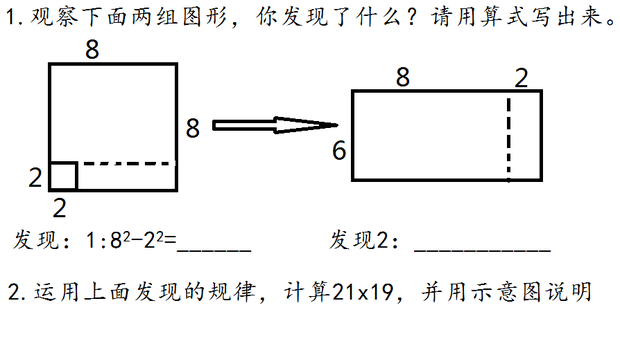# 观察下面两组图形，你发现了什么？请用算式写出来。https://www.teaku.com/2793/1578891501611737.html

## 网友评论

• 公式：

a²-b²=(a+b)(a-b)

21×19

=(20+1)×(20-1)

=20²-1²

=400-1

=399

• 1.64-4=60     （8+2）*（8-2）=10*6=60

2.21*19=（20+1）（20-1）=20*20-1*1=400-1=399

其实就是平方差公式